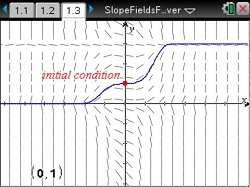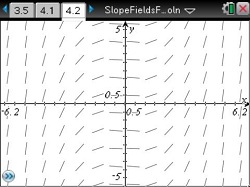# Activities

••• ##### Subject Area

• Math: Calculus: Slope Fields Analysis

• ##### Author9-12

20 Minutes

• ##### Device
•TI-Nspire™ CX/CX II
•TI-Nspire™ CX CAS/CX II CAS
• TI-Nspire™ Navigator™
• TI-Nspire™ CAS
• ##### Software

TI-Nspire™ CAS

3.2

• ##### Report an Issue

Slope Fields Forever

#### Activity Overview

Dynamically explore a particular solution to a differential equation for different initial conditions and investigate slope fields.

#### Key Steps

•In Part 1, students will dynamically explore a particular solution to a differential equation for different initial conditions. Slope fields are investigated. Self-check multiple-choice questions help students build the foundation for the Differential Equation matching activity.

•In the DE matching activity students will use the strategies they have developed from the self-check question to identifying the slope field that corresponds to a differential equation.

The teacher document contains a list of five strategies that can be used to reinforce what the students have discovered.

•In the extension, students explore the slope of the solution to various differential equations to see that it corresponds to the neighboring slope field line segments. Teachers give the instructions of using the deSolve command to find the general solution to the differential equation. This solution is modified for the initial condition and the particular solution can be dynamically changed by grabbing and moving (icx,icy) as in Part 1.